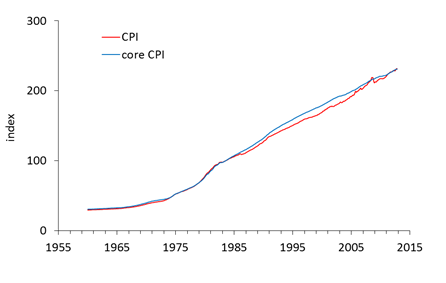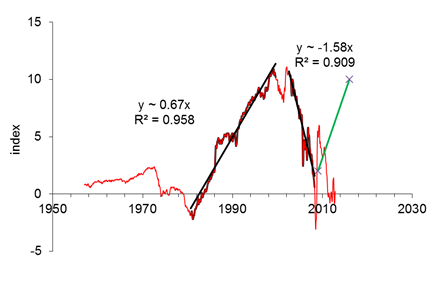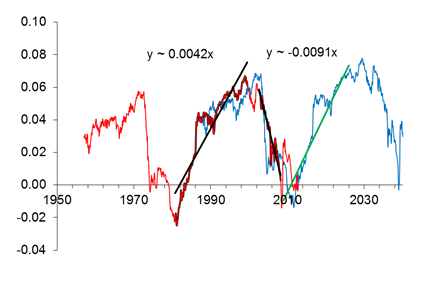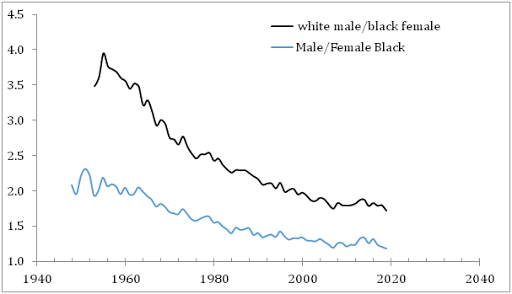## 12/23/12

### Sustainable trends and periodicity in consumer price indices indicate that the era of low energy prices is approaching

Our new paper on CPI and core CPI is now available via MPRA.

Abstract
Five years ago, we found three distinct periods characterized by sustainable quasi-linear trends in the difference between the headline consumer price index (CPI) and the core CPI in the USA. Then we revealed similar behavior in the differences between the CPI and indices of various consumer expenditure categories. We estimated the duration of these trends which varies in a wide range from 8 years to more than 20 years. The transition periods to new trends span shorter intervals of 2 to 5 years. The transition is characterized by a higher level of volatility in the studied CPI differences. In this study, we revisit the revealed trends and transition periods using additional CPI estimates between 2008 and 2012. It is found that our major predictions are right: the headline CPI intersected the core CPI in 2011 and both variables have been evolving in sync since then. The difference between the core CPI and the index of energy has been suffering a transition period since 2008 with extremely high fluctuations. The index of food has been growing relative to the core CPI and did not reach its turning point. The difference between the core CPI and the index of housing passed through its transition period in 2008. When normalized to the CPI, the differences associated with energy and housing demonstrates clear periodicity. The observed trends and periodicity allow predicting the evolution of energy, food, and housing at a horizon of twenty five and more years. The consumer price index of energy may fall in 2013 by ten to twenty per cent from the 2012 level. The periodicity of the related normalized difference implies that this fall may extend into the second half of the 2020s. The housing index will be also falling relative to the core CPI, partly due to its energy related components. It is not excluded that the food price index will be rising another five to ten years. The secular fall in energy and housing prices may induce a lengthy period of very low inflation.

## 12/22/12

### The Headline And The Core Consumer Price Index

The US economy is likely approaching a period of very low, if not negative, price inflation. Our analysis shows that the consumer price index, CPI, will lag behind the core CPI. Deflation is dark time for investors, as the case of Japan unambiguously demonstrates.

The core CPI is often used as a reliable estimate of inflation trends over longer time horizons. It is a well-known fact that the monthly CPI readings reported by the BLS are highly volatile and may give misleading signals on the long-term trends. Usually the prices of food and energy are excluded from the headline CPI in order to obtain a smoother time series. The food and energy prices are considered as having higher volatilities which are responsible for the largest fluctuations observed in the CPI. Therefore, it is implied that the core CPI just misses a stochastic process with a large standard deviation. However, in the long ran the relative evolution of the core and headline CPI is more informative than a simple stochastic time series.

Figure 1 illustrates the evolution of the measured CPI and core CPI in the USA after 1957. Unfortunately, no estimates of the core CPI are available before 1957. Both indices are for all urban customers and seasonally adjusted. In Figure 1, one can observe that the curves are very close before 1980 - the core CPI practically evolved in sync with the CPI. After 1981, the curves diverge with the core CPI growing faster than the CPI. The gap between the indices widens through 2000, and then the curves start to converge and coalesce in the first quarter of 2011.Figure 1. The evolution of the headline and core CPI in the USA.

A better view on the periods of the CPI and core CPI divergence and convergence is represented by the difference between these variables, as displayed in Figure 2. The difference is apparently characterized by the presence of three distinct segments of quasi-linear trend and two short transition periods. Between 1960 and 1978, the difference is relatively stable and varies in a narrow range around +1 unit of index. Between 1979 and 1982, the curve falls to -2 and then suddenly changes its direction. From 1982 to 1999, the core CPI is growing consistently faster and a gap of about 10 units is created. The curve between 1982 and 1999 is well approximated by a straight line with small-amplitude deviations; the latter might be associated with measurement noise. The slope of the linear regression line presented in Figure 2 is -0.7 with R2=0.96. In other words, during these eighteen years the headline CPI was growing by 0.7 units of index faster than the core CPI.

There was another period of high volatility between 1999 and 2002 similar to that observed between 1979 and 1982. The gap reached its peak value two times - in 1999 and in 2003. Since 2003, the gap has been closing in line with a faster growing headline CPI, i.e. the food and energy prices had higher rates of growth. Nevertheless, the CPI was consistently below the core CPI, as Figure 1 demonstrates. In 2008, we extrapolated the observed rate of convergence between the CPI and core CPI and estimated the intercept time somewhere between 2009 and 2010 (here we ignore a short term spike in oil prices in 2008). This negative linear trend was also very reliable with R2=0.91. The convergence was faster - approximately 1.6 units of index per year.

When extrapolated the structure of the difference into the future, we suggested that it would be increasing during the next 8 years as a mirror reflection of the trend observed between 2002 and 2008. The projected trend is shown by green line in Figure 2. Since 2008, the actual curve was above and below the green line. Seemingly, we have missed an important feature of the difference which is best seen in a different representation.Figure 2. The difference between the core and headline CPI.

Figure 3 displays the studied difference normalized to the headline CPI. The pattern is essentially the same as in Figure 2, except the fall in the 1970s, which now has a similar amplitude and duration as the fall between 2003 and 2008. The slope change is almost negligible: from 0.42 in Figure 2 to 0.46 in Figure 3. A striking new feature is the curve self-similarity, which is clear when we shifted the original curve by 30 years ahead (blue line). In other words, the normalized difference is periodic with a period of 30 years. The periodicity suggests a different future, as expressed by a green line with a slope 0.0042. This quasi-linear trend extends into the late 2020s.

In 2012, the normalized curve in Figure 3 returned to the trend. It is highly probable that the rate of CPI growth will fall below that of the core CPI. Equivalently, the joint input of food and energy in the overall price inflation will be declining with time. However, the trajectories of food and energy price evolution are not necessary to be the same.Figure 3. The evolution of the difference between core and headline CPI normalized to the headline CPI. Blue line represents the original curve shifted by 30 years ahead. The new trend is shown by green line.

If to assume that the evolution of the core and headline CPI is driven by independent stochastic processes like random walks, then the deviation between these indices would be a stochastic process itself. From Figure 3, it is more reasonable to assume, however, that there is a tight link between these variables, which provides the observed repeated shape of the normalized difference. For purely stochastic and independent variables such a periodic behavior is highly unlikely.

There are several simple conclusions from the existence of periods and linear trends in the difference between the CPI and core CPI. Firstly, the consumer prices of food and energy are driven by forces which are independent on those behind other goods and services. Secondly, there are breaks in these forces, when the direction of action reverses. Thirdly, one can extrapolate the evolution of the difference between the CPI and core CPI at a horizon of about twenty five years. Fourthly, there exist relatively short transition periods. These periods are characterized by an elevated volatility.

## 12/18/12

### The era of low energy prices

The long-term evolution of energy prices affects the fundamental environment for stock prices. When consumer prices for various goods and services have different but sustainable trends relative to energy prices an opportunity arises for sound investments. Our observations show that some of these sustainable trends have clear turning points which provide investors with invaluable information on buy/sell decision. In this article, we investigate the past and future evolution of the consumer price index (CPI) of energy and demonstrate that it may fall sharply in the near future.

Four years ago we published a paper on the presence of long-term sustainable trends in the differences between various components of the CPI in the USA. We started with the difference between the core CPI (i.e., the CPI less food and energy) and the overall CPI. Then the consumer price index of energy, which gives approximately 9% of the headline CPI, was analyzed. In the beginning of 2008, we tentatively identified a turning point in the difference between the CPI and the energy index and predicted energy prices to fall relative to the core CPI through the first half of the 2010s. Here we revisit this prediction and demonstrate the turning point timing and the duration was relatively accurate.

Here we study the relative evolution of the core consumer price index (CPI) and the CPI of energy. Figure 1 displays the difference between the core CPI and the index for energy for the period between 1960 and 2012. All CPIs are seasonally adjusted and borrowed from the BLS.  Before 1980, these two indices had been growing almost in sync with fluctuation around 10 units of price index. Between 1981 and 1999, the difference grew from -10 to almost 80 units. Between 2001 and 2008, a period of intensive growth in the energy index was observed. Qualitatively, one can distinguish three periods of linear trend and three turning periods with a higher volatility. The last turning point was in 2008 and the index of energy is likely on a declining path relative to the core CPI.  However, the extremely high volatility masks the new trend in the difference.

Figure 1. The difference between the core CPI and the index for energy between 1960 and 2012. There are three periods of linear trend and three turning periods. The most recent turning point was in 2008.

Figure 2 provides a detailed view of the most recent period. The energy index grew much faster than the core CPI between 2001 and 2008. Linear regression gives a slope of -14 for the difference curve. This assumes that the energy index grew by 14 units faster every year than the core CPI.  Since August 2005, the energy price volatility has been at an elevated level and one can likely classify the past seven years as a period of bifurcation. Currently, there is no clear indication of the direction and slope of the next linear trend, however. At the same time, the consumer price index of energy likely reached its peak value in 2007. We expect no further increase in oil price beyond that dictated by the overall price increase. We also expect the current volatility period is close to its natural end  and the difference of the core and energy CPI will be growing along the new trend shown by green line in Figure 2.

Figure 2. Same as in Figure 1, for the period after 2002. Linear trends are shown.

We used only absolute difference so far. It is instructive to analyze the difference in relative terms and we have normalized the difference to the core CPI. Figure 3 illustrates the new pattern. In contrast to Figure 1, the amplitudes and periods of long term fluctuations are similar and the overall evolution seems to be repeatable. Figure 4 exercises the assumption of repeatability. We have shifted the original curve by 27 years ahead and obtained a striking similarity in the amplitude and timing of the energy price falls and rises. Figure 5 shows a detailed picture. From the red curve, one can expect an energy cliff any time soon, as it was observed 27 years ago. The energy index may return to the long term sustainable trend stretching into the 2020s. The era of low energy prices is coming.

Figure 3. The difference between the core and energy CPIs normalized to the core CPI.

Figure 4. Same as in Figure 3 with red curve representing the original (black) curve shifted 27 years ahead.

Figure 5. An energy cliff is coming?

### The mean income gap between white males and black females grows during the democratic presidencies

Two days ago, we compared the mean income evolution of the white and black population and demonstrated that the difference did not change mu...